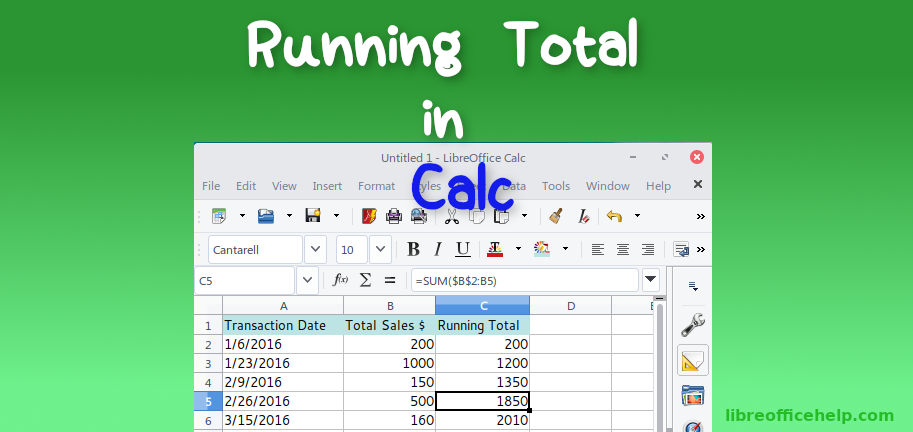# Create Running Total Column in LibreOffice Calc

This short tutorial will explain how to create running total column in LibreOffice Calc.

Running total is a concept of summing data items from the beginning to till current stage. Running total can provide you glimpse of total values as the data sets grows. It mostly useful for may use cases, e.g. to track the total sales value till date, to track current account balance considering withdrawal and deposits and so on.

It is very easy to implement in LibreOffice Calc. Here’s how you can do it.

## Create Running Total Column in Calc

• We have a data series as below where B column is having total sales \$ with dates. We would create running total per day in Column C.
• In cell C2 enter formula =B2. This would ensure that first value remains as the same value of B2.
• In cell C3, enter below formula and press enter. Drag the fill handle till your data sets exists. \$B\$2 is the absolute reference which means it always refers to cell B2 even if formula changes when we drag the fill handle. B3 refers to relative reference, i.e. it changes as per current row number.

=SUM(\$B\$2:B3)

• You can see the running total is calculated.

## Case Study: 1

Based on comment

What if in above example, for some days, total sales is \$0. In those cases, if you apply above formula in Col C, identical running total would be calculated for empty total sales – see below.

So, to overcome that, use a quick IF clause as below to check whether corresponding row is having non zero value and then calculate running total, otherwise skip it. Enter below in cell C3:

=IF(B3>0,SUM(\$B\$2:B3),””)

You can see the total is not calculated for C4 and C5 because B4 and B5 do not have any sales.

## Summary

This is how you can implement quick running total in Calc. You can extend this formula in different cases to achieve running total.

Drop a comment below if you have any questions. If you like this tutorial, take a minute to share it using buttons below. Also follow us/subscribe for tips and tutorials in LibreOffice .

#### Looking for something else?

Read our complete tutorial index of Calc, Writer, Impress and Draw.## Related#### arindam

IT professional by profession and founder of libreofficehelp.com. Loving Linux and other technologies since 2002. I believe in open source and its philosophy. Follow me on Twitter or email me.

## 4 thoughts to “Create Running Total Column in LibreOffice Calc”

1.luvr says:

Alternatively, you could type the following formula in cell C3:

=(C2+B3)

1.arindam says:

Yes, correct. That’s another way of doing it.

2.Steve says:

Is there any way you can enter the formulae for (using the above example) column C, but only display a value in column C if there is an entry for that row? Otherwise it seems every time you create an entry you would have to extend or enter a new formula, otherwise you would have a bunch of identical running totals extending beyond the end of the useful document.

1.arindam says:

Yes. You are correct. (in above example) if say, total sales is empty – the identical running total would be calculated in all empty row. But it is possible to remove that. See updated article above at the end. Thanks for commenting.

This site uses Akismet to reduce spam. Learn how your comment data is processed.